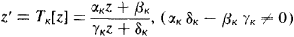Automorphic Function

Automorphic Function

in mathematics, an analytic function whose values do not change if its argument is subjected to certain linear fractional transformations. Automorphic functions include periodic functions and, in particular, elliptic functions.

For example, if the transformations are integer-valued and have the form of z’ = z + ω, where ω is a nonzero complex number, we obtain automorphic functions characterized by the equation f (z + ω) = f (z)—that is, periodic functions with period ω. In this case the transformation which does not modify the function is a shift of the plane by the vector ω. Obviously, the same shift repeated an arbitrary number of times will likewise not change the function. As a result we obtain a group of linear transformations z’ = z + n ω (n = 0, ±1, ±2, . . . ) which do not modify f (z). In the general case, assume that Γ is a group of linear fractional transformationsand that G is a region which is mapped onto itself by each of these transformations. Then a function f which is single-valued and analytic in G is an automorphic function, with respect to the group Γ, if f[TK (z)] = f (z) for k= 1, 2, . . . . The most important case is the one in which G is a circle or half-plane. Such a region may be regarded as the image of a Lobachevskii plane, and the transformations of Γ may be treated as motions in that plane. The corresponding automorphic functions may be regarded as such a generalization of periodic functions when shifts in a Euclidean plane are replaced by motions in a Lobachevskii plane. This point of view, developed by Poincaré, was the cornerstone of the successful construction of a general theory of automorphic functions. (Prior to Poincaré, important results in this field had been achieved by F. Klein.) In general, the entire theory of automorphic functions at present is a remarkable example of the fruitfulness of Lobachevskii’s geometrical concepts as applied to mathematical analysis and the theory of functions.

Besides problems of conformal mapping, other approaches to general automorphic functions are the theory of linear differential equations, the study of algebraic curves of order higher than the fourth, the solution of algebraic equations (for instance, the solution of general fifth-degree equations with one unknown involves automorphic functions), and others.

REFERENCES

Ford, L. R. Avtomorfnye funktsii. Moscow-Leningrad, 1936. (Translated from English.)
Klein, F. Lektsii o razvitii matematiki v 19 stoletii, part 1. Moscow-Leningrad, 1937. Chapter 8. (Translated from German.)
Golubev, V. V. Lektsii po analiticheskoi teorii differentsial’nykh uravnenii, 2nd ed. Moscow-Leningrad, 1950.
Golubev, V. V. Odnoznachnye analiticheskie funktsii: Avtomorfnye funktsii. Moscow, 1961.
References in periodicals archive ?
The range of an almost automorphic function is relatively compact on X and hence is bounded.
A classical example of an almost automorphic function, which is not almost periodic is the function defined by
It discusses discontinuous groups through the classical method of Poincare and the model of the hyperbolic plane; develops automorphic functions and forms through the Poincare series, with discussion of formulas for divisors of a function and form; and details the connection between automorphic function theory and Riemann surface theory, as well as applications of Riemann-Roch theorem.
They cover scattering theory, automorphic functions, field theory, the theory of solitons, quantum groups, and knots.
the weighted pseudo almost periodic functions on time scales are a particular case of the weighted pseudo almost automorphic functions on time scales) and some of the results in [U.
Notice that it is also possible to consider E = PAP(R, C), AA(R, C), AAA(R, C) or PAA(R, C), namely, the sets of pseudo-almost periodic functions, almost automorphic functions, asymptotically almost automorphic functions and pseudo-almost automorphic functions respectively, see for instance [4,5,9,10,24, 27, 30, 33].
The major themes addressed are hyperbolic geometry, automorphic functions, and applications to number theory; ordinary differential equations and dynamical systems; celestial mechanics and related problems; functions of several complex variables; algebraic topology and the Poincare conjecture; partial differential equations of mathematical physics; probability; continuous groups (Lie groups) and their algebras; the principle of relativity and the Poincare group; applied physics; and the philosophy of science.
BURNSIDE, On a class of automorphic functions, Proc.
Generalized Functions; Volume 6: Representation Theory and Automorphic Functions (reprint, 1969)
Topics discussed include Faltings' finiteness theorem, Wiles' proof of Fermat's Last Theorem, modular curves and parametrizations, Heegner points, Shimura varieties, the minimal model program, moduli spaces of curves and maps, deformation theory, Galois cohomology, harmonic analysis, and automorphic functions.